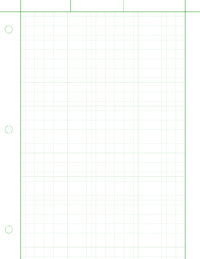# Graph paper

Graph paper

Graph paper, graphing paper, grid paper or millimeter paper is writing paper that is printed with fine lines making up a regular grid. The lines are often used as guides for plotting mathematical functions or experimental data and drawing diagrams. It is commonly found in mathematics and engineering education settings and in laboratory notebooks. Graph paper is available either as loose leaf paper or bound in notebooks. It is becoming less common as computer software such as spreadsheets and plotting software has supplanted many of the former uses of graph paper.[citation needed] Some users of graph paper now print pdf images of the grid pattern as needed rather than buying it pre-printed. There are websites that will generate pdfs of graph paper to the user's specifications.

## Formats

• Quad paper is a common form of graph paper with a sparse grid printed in light blue or gray and right to the edge of the paper. This is often four squares to the inch for work not needing too much detail. It is sometimes referred to as quadrille paper.
•Engineering paper
Engineering paper is traditionally printed on light green or tan translucent paper. The grid lines are printed on the back side of each page and show through faintly to the front side. Each page has an unprinted margin. When photocopied or scanned, the grid lines typically do not show up in the resulting copy, which often gives the work a neat, uncluttered appearance. In the US and Canada, some engineering professors require student homework to be completed on engineering paper.
• Genkō yōshi – A type of manuscript paper used in Japan, normally printed with 400 squares in two sets of 20 lines of 10, used for compositions written in horizontal script.
• Hexagonal paper shows regular hexagons instead of squares. These can be used to map geometric tiled or tesselated designs among other uses
• Isometric graph paper or 3D graph paper is a triangular graph paper which uses a series of three guidelines forming a 60° grid of small triangles. The triangles are arranged in groups of six to make hexagons. The name suggests the use for isometric views or pseudo-three dimensional views. Among other functions, they can be used in the design of trianglepoint embroidery.
• Logarithmic paper has rectangles drawn in varying widths corresponding to logarithmic scales for semilog graphs or log-log graphs.
• Normal probability paper is another graph paper with rectangles of variable widths. It is designed so that "the graph of the normal distribution function is represented on it by a straight line".
• Polar coordinate paper has concentric circles divided into small arcs or 'pie wedges' to allow plotting in polar coordinates.

In general, graphs showing grids are sometimes called Cartesian graphs because the square can be used to map measurements onto a Cartesian (x vs. y) coordinate system. It is also available without lines but with dots at the positions where the lines would intersect.

Wikimedia Foundation. 2010.

### Look at other dictionaries:

• graph paper — UK US noun [U] (also graphing paper) GRAPHS & CHARTS ► special paper with small squares on it, which is used for drawing plans or graphs: »He quickly charted the spending curve on graph paper …   Financial and business terms

• graph paper — graph .paper n [U] paper with many squares printed on it, used for drawing ↑graphs …   Dictionary of contemporary English

• graph paper — graph ,paper noun uncount paper that has small squares printed on it, used for drawing GRAPHS …   Usage of the words and phrases in modern English

• graph paper — ► NOUN ▪ paper printed with a network of small squares to assist the drawing of graphs or other diagrams …   English terms dictionary

• graph paper — n. paper with small ruled squares on which to draw graphs, diagrams, etc …   English World dictionary

• graph paper — noun paper that has lines to permit drawing graphs • Hypernyms: ↑paper * * * noun, pl ⋯ ers [count] : paper that is covered with squares formed by lines that run from top to bottom and side to side * * * ˈgraph paper [graph paper] noun …   Useful english dictionary

• graph paper — N UNCOUNT Graph paper is paper that has small squares printed on it so that you can use it for drawing graphs …   English dictionary

• graph paper — graph′ pa per n. pri paper printed with a pattern of lines for plotting graphs • Etymology: 1925–30 …   From formal English to slang

• graph paper — paper printed with a pattern of straight or curved lines, esp. a grid of small squares, for plotting or drawing graphs and curves. [1925 30] * * * …   Universalium

• graph paper — paper ruled with horizontal and vertical lines for drawing graphs …   English contemporary dictionary